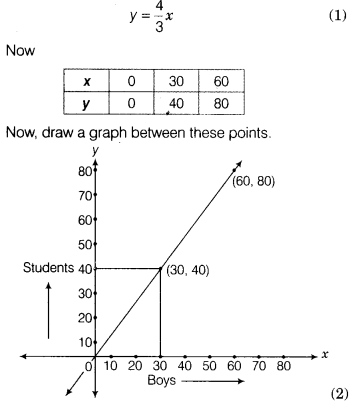# Set up an equation between the students of a class and boys and then draw its graph

The ratio of girls and boys in a class is 1 : 3. Set up an equation between the students of a class and boys and then draw its graph. Also find the number of boys in a class of 40 students from the graph.

Total students in the class=y
Let the boys in the class=x, then equation between the students and the boysFrom the graph it is clear that there are 30 boys in a class of 40 students.